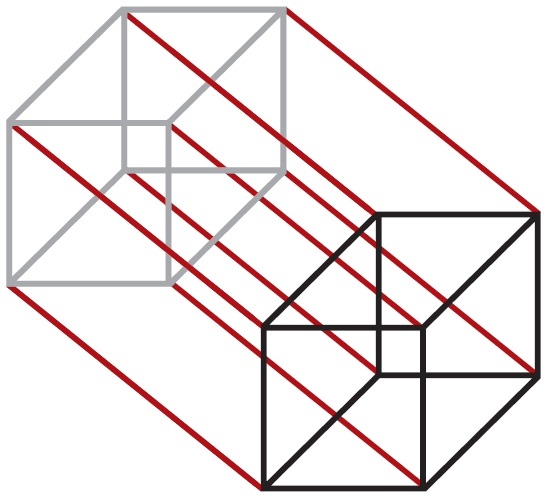# Q: What's in a Logo? A: Mathematics

by Tom Hagelskjær on 02. February 2022

Maybe you have wondered where our logo comes from and what it actually means. If you have, we hope the following will answer these questions.

Just as our name suggests, mathematics is the strong foundation on which our company has been built. The same applies to our logo.

The Cryptomathic logo is a 4D (4-dimensional) cube projected onto a 2D (2-dimensional) plane, with one 3D (3-dimensional) cube highlighted. However, as terrestrial beings, we can only see things in 3D, so it is hard to visualise something with more dimensions. The 4D cube is obtained by taking a (shadow) copy of the highlighted 3D cube, and joining all the corresponding corners of the two 3D cubes.At Cryptomathic's previous anniversary, a mathematical challenge was put forward to all employees, centred on our logo. The challenge was to answer the following 3 questions:

• How many cubes are contained in our logo?
• How many squares are contained in our logo?
• How many edges are contained in our logo?

We only had about 1 minute (really!) to write down our answers, so basically had to guess, and in fact nobody managed to get all three answers correct. This lead on to an even bigger challenge for some of us.  For the rest of the day (and some of the next day) we devoted much of our time to deriving formulas for calculating these numbers - of course without cheating by looking at textbooks!

As it is very difficult to imagine things in four (or more) dimensions, it's often an advantage to re-formulate such questions using mathematical notation, and also to refer to the question in more general terms. Thus, we'll generalise into looking at cubes of n dimensions, and ask how many sub-cubes of k dimensions are contained within these, for 0 ≤ k ≤ n.

The cube of n dimensions (the n-cube) is defined mathematically by its corners:

x = (x1, x2, …, xn),  for  xi ε {0, 1}.

A sub-cube of k dimensions (a k-cube contained in the n-cube) is defined by fixing n-k of the xi's to chosen values, while letting the k remaining xi's vary. For example, a 2-cube (square) on the 3-cube (cube) is given by fixing one xi, and a 1-cube (edge) is given by fixing two xi's. Actually, it is the 1-cubes (edges) that are usually drawn when trying to picture an n-cube.

Obviously, there are 2n 0-cubes (corner points) in the n-cube, and only 1 n-cube (the n-cube itself). The argument below shows that in general the number of k-cubes contained in the n-cube is given by:

fn,k = 2n-k × (nk

where (nk)  is the usual combinatorial function for selecting k out of n possibilities, given by:

(nk) = n! / k!×(n-k)!

The argument goes as follows:

• There are 2n corners in the n-cube.
• Each corner has (nk) k-cubes touching it (choosing which k xi's to vary)
• Each k-cube touches 2k corners

Multiplying the first two of these counts each k-cube 2k times, thus giving the result above by dividing this out. A similar argument would just say that we could choose the n-k xi's to fix in (nk) ways, and then choose the actual values of these in 2n-k ways.

Alternatively, if one is very good at imagining things in n dimensions the result can also be found by observing that generally the n-cube can be constructed from the (n-1)-cube by taking a (shadow) copy of it and then combining all corresponding corners in the two copies. Besides from doubling all contained k-cubes this also gives an additional k-cube for each (k-1)-cube in the original (n-1)-cube. Combined with the previous observations for k = 0 and k = n, this gives the recursive formulas:

 fn,k = 1 ,  for  k = n fn,k = 2n ,  for  k = 0 fn,k = 2 × fn-1,k + fn-1,k-1 ,  for  0 < k < n

The formula for fn,k derived earlier can easily be proved to be the unique solution to these equations.

The answer to the original challenge can now be calculated as:

f4,3 = 21 × (43) = 8

f4,2 = 22 × (42) = 24

f4,1 = 23 × (41) = 32

Image: "Nixie numbers", courtesy of Lenore Edman, Flickr (CC BY 2.0)

## Want to know how we can help ?

Get in touch to better understand how our solutions secure ecommerce and billions of transactions worldwide.